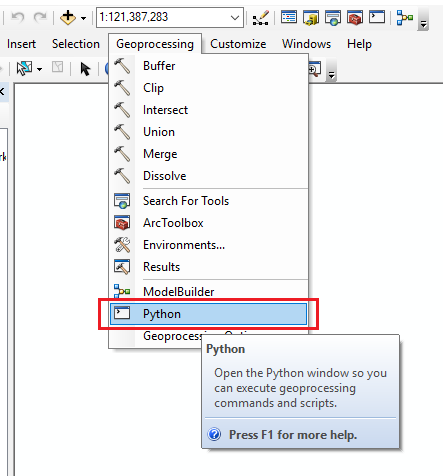HOW TO

# Print the spatial reference of feature classes using Python script

## Summary

A spatial reference is the coordinate system used to store the location of each feature class and raster dataset, as well as other coordinate properties such as the coordinate resolution for x,y coordinates, and optional z and m coordinates. For more information about spatial references, refer to the following web help document, ArcGIS Desktop: An overview of spatial references.

Retrieving multiple spatial references can take a long time. As an alternative, the feature class spatial reference can be retrieved by using the ArcPy function, arcpy.Describe() and the spatialReference ArcPy class. The script can be modified to use loop expressions to iterate between all existing feature classes in a file geodatabase.

## Procedure

The following steps demonstrate how to iterate between feature classes and print spatial reference of feature classes using a Python script:

1. In ArcMap, click the Geoprocessing tab and select Python. This opens the Python console.1. Import the ArcPy module and set the environment workspace.
```import arcpy

arcpy.env.workspace = r"[WORKSPACE_NAME]"```
```Note:
To use the current default workspace, replace the environmental workspace parameter as follows:

arcpy.env.workspace = arcpy.GetParameterAsText(0)```
1. Retrieve the list of feature classes in the input folder.
```feature_classes = arcpy.ListFeatureClasses()
print feature_classes```
1. Iterate between feature classes and retrieve the spatial reference by creating an object.
```for fc in feature_classes:
spatial_ref = arcpy.Describe(fc).spatialReference```
1. Create a selection statement to differentiate between unknown and defined spatial reference, and print the spatial reference. The result displays the list of spatial reference of all feature classes in the designated workspace.
```if spatial_ref.name == "Unknown":
print("{0} has an unknown spatial reference".format(fc))

else:
print("{0} : {1}".format(fc, spatial_ref.name))```

The following script is an example of a full working code:
```import arcpy

# Set the workspace environment
arcpy.env.workspace = arcpy.GetParameterAsText(0)

# Get a list of the feature classes in the input folder
feature_classes = arcpy.ListFeatureClasses()
print feature_classes

### Loop through the list

for fc in feature_classes:
# Create the spatial reference object
spatial_ref = arcpy.Describe(fc).spatialReference

# If the spatial reference is unknown
if spatial_ref.name == "Unknown":
print("{0} has an unknown spatial reference".format(fc))

# Otherwise, print out the feature class name and
# spatial reference
else:
print("{0} : {1}".format(fc, spatial_ref.name))```

Article ID:000018478

Software:
• ArcMap

Get help from ArcGIS experts

Contact technical support